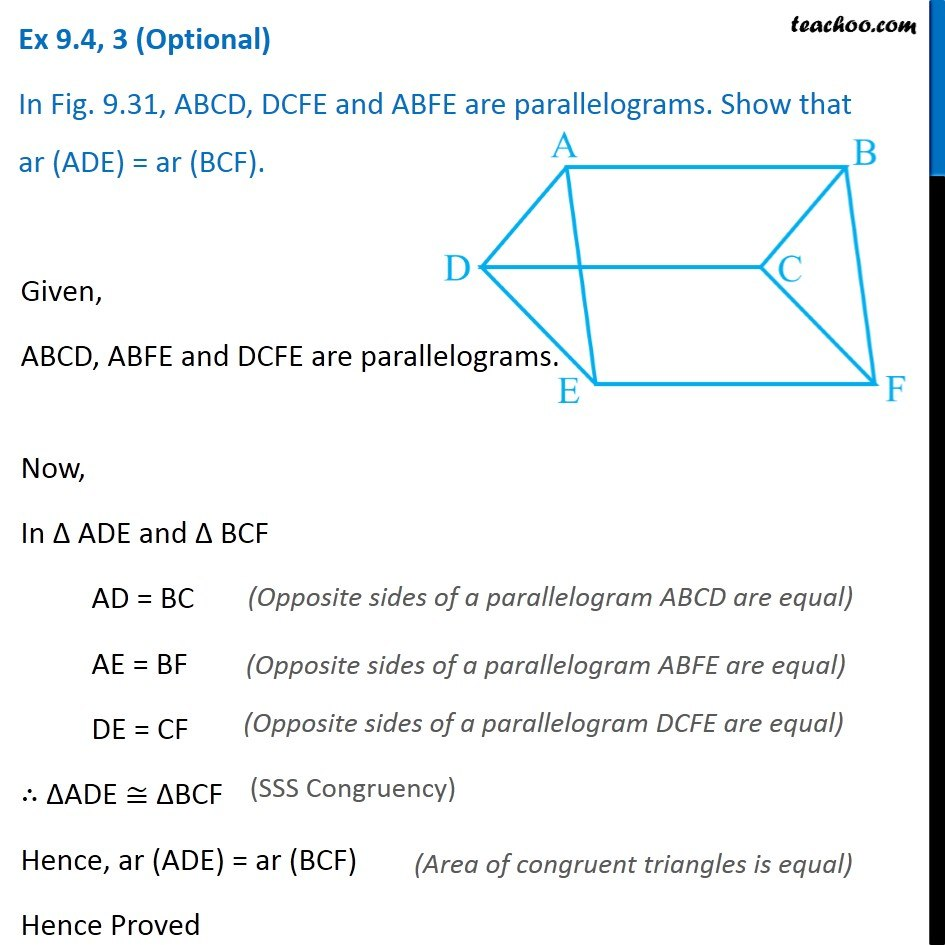Important questions on Parallelograms and Triangles

Areas of Parallelograms and Triangles
Serial order wiseLearn in your speed, with individual attention - Teachoo Maths 1-on-1 Class

### Transcript

Question 3 In Fig. 9.31, ABCD, DCFE and ABFE are parallelograms. Show that ar (ADE) = ar (BCF). Given, ABCD, ABFE and DCFE are parallelograms. Now, In ∆ ADE and ∆ BCF AD = BC AE = BF DE = CF ∴ ∆ADE ≅ ∆BCF Hence, ar (ADE) = ar (BCF) Hence Proved (Opposite sides of a parallelogram ABCD are equal) (Opposite sides of a parallelogram ABFE are equal) (Opposite sides of a parallelogram DCFE are equal) (SSS Congruency) (Area of congruent triangles is equal)